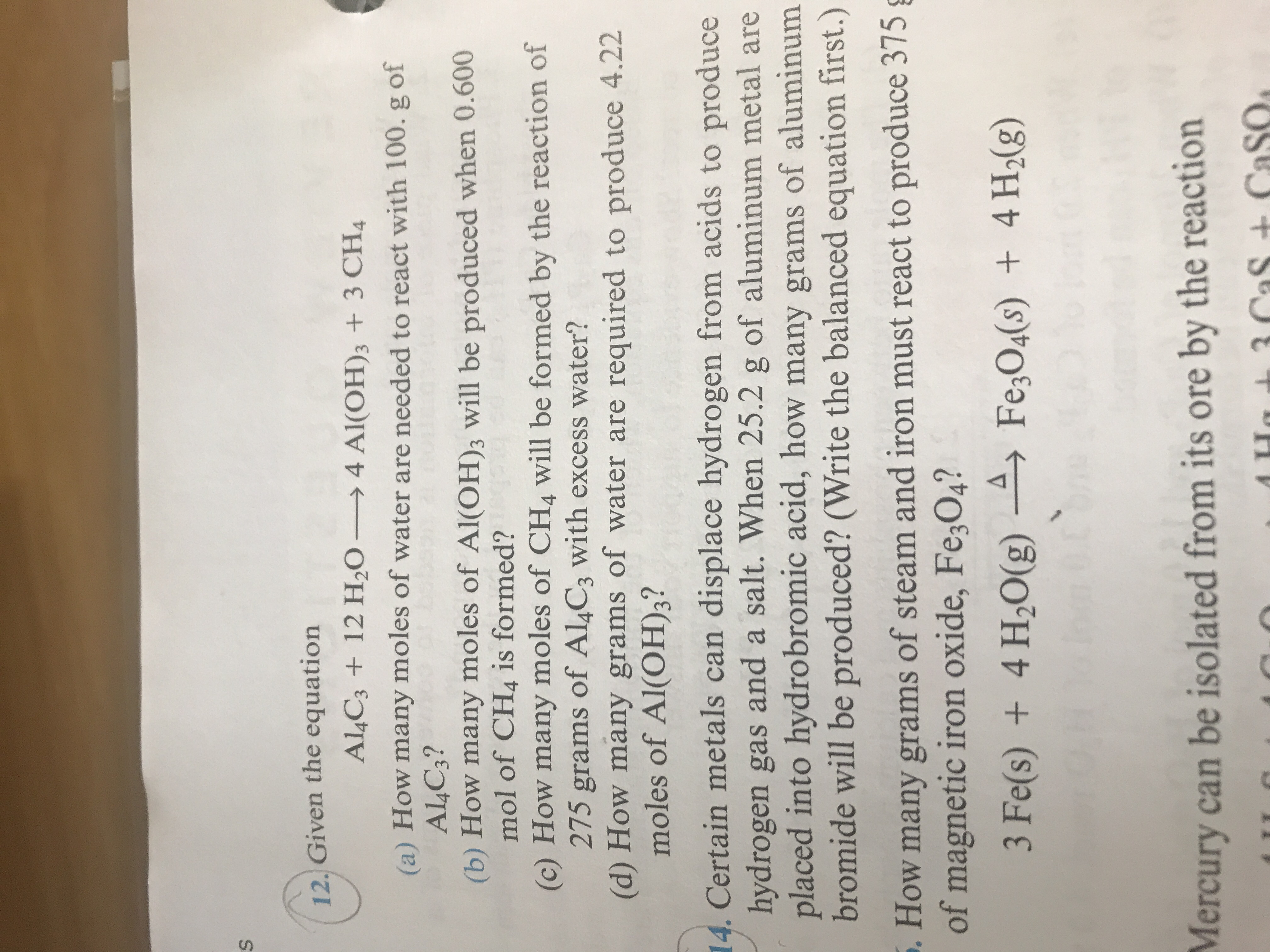# 12, Given the equation Al,C3 + 12 H2O-→ 4 Al(OH)3 + 3 CH, (a) How many moles of water are needed to react with 100. g of (b) How many moles of Al(OH)s will be produced when 0.600 (c) How many moles of CH4 will be formed by the reaction of (d) How many grams of water are required to produce 4.22 A4C3? mol of CH4 is formed? 275 grams of Al4C3 with excess water? moles of Al(OH)3? Certain metals can displace hydrogen from acids to produce hydrogen gas and a salt. When 25.2 g of aluminum metal are placed into hydrobromic acid, how many grams of aluminum bromide will be produced? (Write the balanced equation first.) 14. . How many grams of steam and iron must react to produce 375 of magnetic iron oxide, Fe,04? Mercury can be isolated from its ore by the reaction

Question

On question B can you go from moles to moles even if it starts with moles?help_outlineImage Transcriptionclose12, Given the equation Al,C3 + 12 H2O-→ 4 Al(OH)3 + 3 CH, (a) How many moles of water are needed to react with 100. g of (b) How many moles of Al(OH)s will be produced when 0.600 (c) How many moles of CH4 will be formed by the reaction of (d) How many grams of water are required to produce 4.22 A4C3? mol of CH4 is formed? 275 grams of Al4C3 with excess water? moles of Al(OH)3? Certain metals can displace hydrogen from acids to produce hydrogen gas and a salt. When 25.2 g of aluminum metal are placed into hydrobromic acid, how many grams of aluminum bromide will be produced? (Write the balanced equation first.) 14. . How many grams of steam and iron must react to produce 375 of magnetic iron oxide, Fe,04? Mercury can be isolated from its ore by the reaction fullscreen

### Want to see this answer and more?

Step-by-step answers are written by subject experts who are available 24/7. Questions are typically answered in as fast as 30 minutes.*